The OEIS Foundation is supported by donations from users of the OEIS and by a grant from the Simons Foundation.Hints (Greetings from The On-Line Encyclopedia of Integer Sequences!)

## (Page 8)## A Binomial Coefficient Sum

When analyzing the number of steps that a certain algorithm takes to run, you encounter a binomial coefficent sum, perhaps: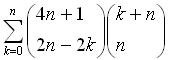You know that there are powerful methods available for automatically simplifying such sums
(see for example the book by M. Petkovsek, H. S. Wilf and D. Zeilberger, A=B, Peters, Wellesley, MA, 1996;
or Doron Zeilberger's web site).

But you are in a hurry, so you start by evaluating the sum for the first few values of n. This produces the sequence

1, 12, 240, 5376, 126720, 3075072, ...

which you can then look up in the OEIS.

One of three things will happen!

• If you are very lucky, the sequence will be there, together with a note that this is a binomial coefficent sum.
• If you are just lucky, the sequence will be there (but without a comment about it being a binomial coefficient sum), in which case you now have a conjectured binomial coefficent identity that you can prove at your leisure by the above-mentioned methods. (You may also use the Contributing a new sequence or comment web page to send a comment to the database.)
• If the sequence is not there, you can use the same web page to send the sequence, the summmation, and anything else you know about it to the database. This way the next person who encounters it will be helped, and your name will be preserved in the database!

In this particular example you were very lucky. Here is the reply from the database.### Greetings from the On-Line Encyclopedia of Integer Sequences!

 A006588 4^n*(3*n)!/((2*n)!*n!). +01
 1, 12, 240, 5376, 126720, 3075072, 76038144, 1905131520, 48199827456, 1228623052800, 31504481648640, 811751838842880, 20999667135283200, 545086744471535616, 14189559697354260480, 370298578584748425216 (list)
 OFFSET 0,2 REFERENCES W. A. Whitworth, DCC Exercises in Choice and Chance, Stechert, NY, 1945, p. 35. The right-hand side of a binomial coefficient identity in H. W. Gould, Combinatorial Identities, Morgantown, 1972; Eq. 3.115, page 35. FORMULA Sum_{k=0..n} binomial(4n+1,2n-2k)*binomial(n+k,k) = 4^n*binomial(3n,n). a(n) ~ 1/2*3^(1/2)*pi^(-1/2)*n^(-1/2)*3^(3*n)*{1 - 7/72*n^-1 + ...} - Joe Keane (jgk(AT)jgk.org), Jun 11 2002 MAPLE A006588 := n->add( binomial(4*n+1, 2*n-2*k)*binomial(n+k, k), k=0..n); CROSSREFS Sequence in context: A009052 A012303 A012538 this_sequence A009150 A009080 A002166 Adjacent sequences: A006585 A006586 A006587 this_sequence A006589 A006590 A006591 KEYWORD nonn,easy,nice AUTHOR N. J. A. Sloane (njas(AT)research.att.com)
 page 1Thus your sum turns out to be equal to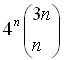That particular sum is just one chosen at random from Gould's table.

The database contains sequences produced by many similar summations.

It would be nice to get more examples, particularly of sums where the answers are rational numbers rather than integers. This would be an excellent project for a volunteer.Click the single right arrow to go to the next demonstration page, or the single left arrow to return to the previous page.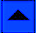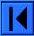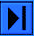Lookup | Welcome | Wiki | Register | Music | Plot 2 | Demos | Index | Browse | More | WebCam
Contribute new seq. or comment | Format | Style Sheet | Transforms | Superseeker | Recent
The OEIS Community | Maintained by The OEIS Foundation Inc.

Last modified September 21 00:35 EDT 2020. Contains 337265 sequences. (Running on oeis4.)